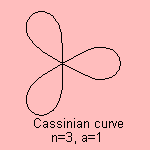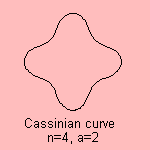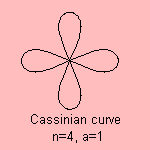# Cassinian curve

## higher

last updated: 2003-07-25It is the curve for which the product of multiple polar radii is constant.
The curve is an algebraic curve of degree 2n.

In the case of a regular polygon the curve can be written in polar coordinates as1)
For a < 1, the curve consists of n pieces.
For a > 1, the curve is just one closed curve.
For a = 1, the curve is a sinusoidal spiral.

For n = 2 we see the Cassinian oval.Think about a magnetic field created by n parallel threads with the same current.
The magnetic field lines in a plane orthogonal to the threads are Cassinian curves for which the foci are the intersections of the threads with the plane.

The Cassinian curve has been studied by Serret (in 1843), and the curve is named after Cassini.

notes

1) In other words: